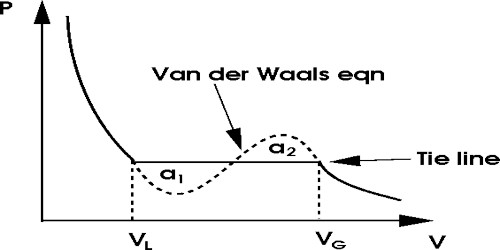Chemistry

# Van der Waals Equation of StatesVan der Waals Equation of States

If the experimental result of different scientists are considered it is found that above critical temperature and at low pressure a gas behaves more or less similar to ideal gas and tends to show the tendency to follow Boyle’s law. But by keeping the gas below the critical temperature if the pressure is continuously increased properties of a gas continue to deviate from Boyle’s Law. Finally, a gas transform into a liquid. From the ideal gas equations for a mole of gas i.e., PV = RT, the behavior of real gas cannot be explained at all. So, it was necessarily realized to derive an equation to satisfy a real gas.

In this connection, scientists Van der Waals and Ham emphasized to make correction in the two postulates of kinetic theory of gases. Finally, taking into considerations and making necessary corrections of the two postulates expressed an equation for real gases. This equation is primarily able to analyze the isothermal graphs.

Taking into consideration of limited shape of gas molecules and intermolecular force between them, be corrected the ideal gas equation in the following way:

(P + a/V2) (V – b) = RT

Here a and b quantities are constant for a particular gas, but not have same values for all gases. Although Van der Waals constant ‘a’ and ‘b’ are called constants but actually they are different not only for different gases but also are different at different temperatures for the same gas. According to this theory this constant b = Vc/3, but in reality, b = Vc/2; Vc = critical angle.